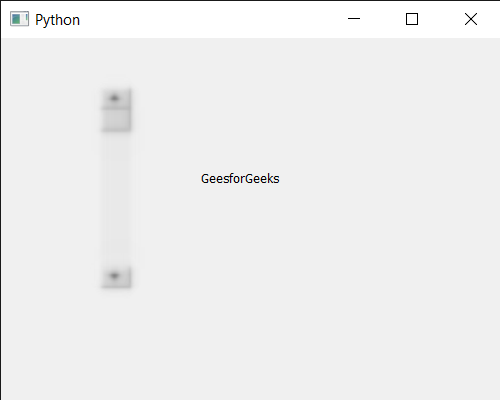GFG App
Open AppBrowser
Continue

# PyQt5 QScrollBar – Setting Graphic Effect to it

In this article we will see how we can set graphic effect to the QScrollBar. QScrollBar is a control that enables the user to access parts of a document that is larger than the widget used to display it. Slider is the scroll-able object inside the bar.The setGraphicsEffect function is for setting the scroll bar’s graphics effect. Sets effect as the widget’s effect. If there already is an effect installed on the scroll bar, QWidget will delete the existing effect before installing the new effect.

In order to do this we will use setGraphicsEffect method with the scroll bar object. Syntax : scroll.setGraphicsEffect(graphics) Argument : It takes QGraphicsEffect object as argument Return : It returns None

Below is the implementation

## Python3

 `# importing libraries` `from` `PyQt5.QtWidgets ``import` `*` `from` `PyQt5 ``import` `QtCore, QtGui` `from` `PyQt5.QtGui ``import` `*` `from` `PyQt5.QtCore ``import` `*` `import` `sys`     `class` `Window(QMainWindow):`   `    ``def` `__init__(``self``):` `        ``super``().__init__()`   `        ``# setting title` `        ``self``.setWindowTitle("Python ")`   `        ``# setting geometry` `        ``self``.setGeometry(``100``, ``100``, ``500``, ``400``)`   `        ``# calling method` `        ``self``.UiComponents()`   `        ``# showing all the widgets` `        ``self``.show()`       `    ``# method for components` `    ``def` `UiComponents(``self``):`   `        ``scroll ``=` `QScrollBar(``self``)`   `        ``# setting geometry of the scroll bar` `        ``scroll.setGeometry(``100``, ``50``, ``30``, ``200``)`   `        ``# making its background color to green` `        ``scroll.setStyleSheet("background : lightgrey;")`   `        ``# QGraphicsEffect` `        ``graphic ``=` `QGraphicsBlurEffect(``self``)`   `        ``# setting graphic effect to the scroll bar` `        ``scroll.setGraphicsEffect(graphic)`   `        ``# creating a label` `        ``label ``=` `QLabel("GeeksforGeeks", ``self``)`   `        ``# setting geometry to the label` `        ``label.setGeometry(``200``, ``100``, ``300``, ``80``)`   `        ``# making label multi line` `        ``label.setWordWrap(``True``)`   `        ``# getting value changed signal` `        ``scroll.valueChanged.connect(``lambda``: do_action())`   `        ``# method called when signal is emitted` `        ``def` `do_action():` `            `  `            ``# setting text to the label` `            ``label.setText("Current Value : " ``+` `str``(scroll.value()))`         `# create pyqt5 app` `App ``=` `QApplication(sys.argv)`   `# create the instance of our Window` `window ``=` `Window()`   `# start the app` `sys.exit(App.``exec``())`

Output :My Personal Notes arrow_drop_up